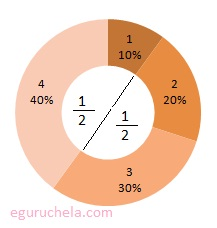# Fraction Operations

When adding or subtracting fractions, we ensure that the fractions being added have the same denominator. If they do not, find the LCD for the fractions and put each in its equivalent form. Then, simply add or subtract the numerators of the fractions. When multiplying fractions, we simply multiply the numerators together and then multiply the denominators together. When you divide two fractions, we take the reciprocal of the second fraction, or bottom fraction, and multiply.